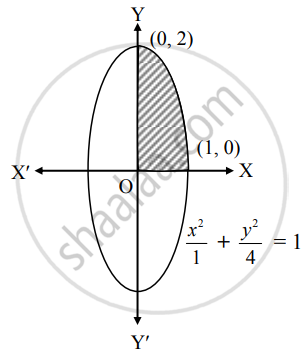# Find the area of the ellipse x21+y24 = 1, in first quadrant - Mathematics and Statistics

Sum

Find the area of the ellipse x^2/1 + y^2/4 = 1, in first quadrant

#### Solution

Given equation of the ellipse is x^2/1 + y^2/4 = 1.

∴ y^2/4 = 1 − x2

∴ y2 = 4(1 – x2)

∴ y = +-  2sqrt(1 - x^2)

∴ y = 2sqrt(1 - x^2)   ......[∵ In first quadrant, y > 0]∴ Required area

=  int_0^1 y  "d"x

= int_0^1 2sqrt(1 - x^2)  "d"x

= 2[x/2 sqrt(1 - x^2) + 1^2/2 sin^-1(x/1)]_0^1

= 2[0 + 1/2 sin^-1 (1) - 0]

= 2[1/2 (pi/2)]

= pi/2 sq.units

Concept: Area Between Two Curves
Is there an error in this question or solution?